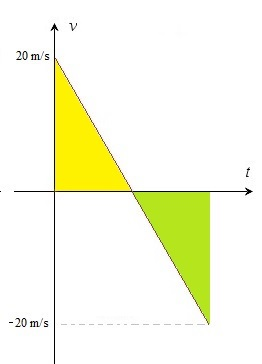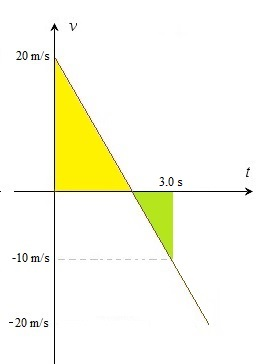# Free fall

Up a level : Pre DP, Mechanics and Electronics
Previous page : Formulas for the displacement, starting at non zero velocity
Next page : To throw a ball - an animationDropping a ball

In this we will assume the air friction, the drag, is zero.

As mentioned earlier the acceleration of a freely falling object, if discard air friction, is about 9.81 m/s2. (Close to the surface of earth at least.). We looked at an example of this in a previous section. There we saw that the distance travelled over an interval was equal to the average of the velocity in the beginning of the interval and the velocity at the end of the interval,  and as we saw in the last section it is exactly what to expect, since, as we found,$s = \frac{{{v_1} + {v_0}}}{2} \cdot t$

for uniform acceleration.

The free fall acceleration is often written as g.  So g=9.81 m/s2 or something close to that depending on where you live.

Throwing a ball

So far the velocity/speed and displacement/distance distinction has not had that much of an impact. But let us look at a  situation where all of this mater. Let us throw a ball straight up in the air with a speed of 20 m/s.  Now, the first decision we need to make is what our positive direction is. The initial  velocity will now be v=20 m/s. (Had we chosen  that down is the positive direction then we would use the velocity –20 m/s.).

Our choice of  direction will give us that the acceleration of gravity is –g, since it acts in the downward direction.  To simplify the calculations in this example we will proximate g by 10 m/s2 .

Let us sketch a v/t-diagram over the situation from when the velocity is 20 m/s until it is 0 m/s.The velocity  is at first 20 m/s. After one second it is 10 m/s since we loose 10 m/s every second. This means that the velocity is 0 m/s after 2 seconds. The ball will now be at its highest point. So how high up in the air is it (from the height we released it at)? The displacement corresponds to the area under the curve. I.e to the area of the triangle. This gives us 2·20/2=20 m.

The acceleration of gravity has thus far been a retardation.

The ball will though continue to accelerate by –10 m/s2 . So from 0 m/s we get to –10 m/s after yet another second, and –20 m/s at the 4 s mark.So what is our displacement now? Think about what the graph represent. We throw a ball straight up in the air and after two seconds it reaches the highest point in its path, where the velocity will be 0 m/s. Then it will fall down back to the starting height, so the displacement is 0 m.

So how to make sense of that given the graph? Rememberer that the area “under” the graph represents the displacement. But is the curve is under the horizontal axis graph, then  we are dealing with the area above the graph. Let us look at a graph of this.The yellow triangle has the area 2·20/2=20 m , as we have already seen.  This is the displacement between the times t=0 s and t=2 s. The object have moved up 20 m to its peak height.

But since the velocity is negative in the end the “height” of the green triangle is –20 m/s.  that would give the green triangle the area 2·(–20)/2= –20 m. These –20 m represent the displacement from  t=2 s and t=4 s. The object has fallen 20 m.

Together those two values would give a total displacement of 20 + (–20) = 0 m.

The total distance travelled is the 20 meters up and then 20 meters down, so in total 40 m. We thus calculate that as the total area of the yellow and green triangle, now counting the green triangle as a positive area since in distance calculations we  don’t care about the direction.

Some other time (some other space)

So what was the height at say t=3 s?  Lets draw a v/t-diagram again.The yellow triangle still represent 20 m.The velocity at the 3 s mark is our original 20 m/s  plus the change in velocity Δv=at =–10·3= –30 m/s. That would give us a velocity of 20–30 = –10 m/s. Another way to see this is that at t=2 s the velocity was 0 m/s, then we accelerate at –10 m/s2 for a second to get a velocity of –10 m/s

The green triangle would thus have

the area = displacement of  –10·1/2 = –5 m.

All in all we would thus  give us a displacement, that in this case is equal to the height =20 – 5= 15 m.

The distance travelled would at that moment be 20 meters up and 5 meters down. That is 20+5=25 m.Up a level : Pre DP, Mechanics and Electronics
Previous page : Formulas for the displacement, starting at non zero velocity
Next page : To throw a ball - an animationLast modified: Feb 11, 2017 @ 09:31